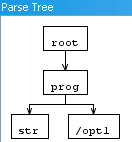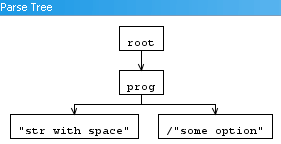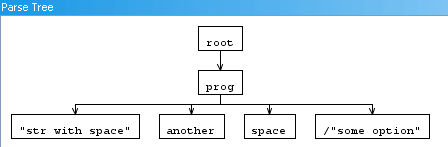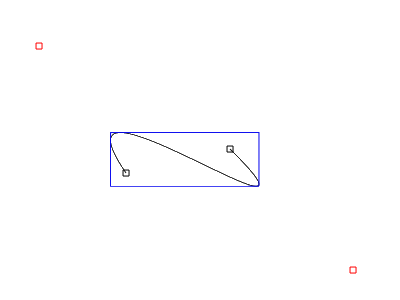## How to install Magento on Server2Go[Versions:
Magento : 1.4.1.1
Server2Go:1.8.1 (includes APACHE 2.2.15;PHP 5.3.2;SQLITE,MYSQL 5.1.46)]

2. Unblock (windows security feature) both zip files, (right click on zip, properties , Unblock)
3. Extract both zip files.
4. Go to the Server2Go folder and start Server2Go ( a broweser will open, IE)
6. Create a database named ‘Magento’ (or any other name, but this howto will use Magento)
7. Close down the browser, You’ll see that Server2Go is shutting down.
8. Rename the ‘htdocs’ to ‘htdocs_org’
9. Create a new folder ‘htdocs’
10. Extract all files in the new ‘htdocs’ folder. Do not place the magento folder in the root but it’s content.

Now we need to edit a few files:

Folder: server2go\
File: pms_config.ini

Change:

(Not required, but recommended)

LocalMirror=1

to

LocalMirror=0

MySQLCmd=–skip-innodb

to

MySQLCmd=

make changes to the [browser] settings if you want.

folder : server2go\server\config_tpl
file: php.ini

Change:

max_execution_time = 30     ; Maximum execution time of each script, in seconds
memory_limit = 128M      ; Maximum amount of memory a script may consume (8MB)

to

max_execution_time = 600     ; Maximum execution time of each script, in seconds
memory_limit = 512M      ; Maximum amount of memory a script may consume (8MB)

;extension=php_curl.dll

to

extension=php_curl.dll

;extension=php_pdo_mysql.dll

to

extension=php_pdo_mysql.dll

Installation of Magento:

1. Start the server2go executable (again IE opens)
2. If magento files ware placed in the correct location, you will see the ‘Welcome to Magento’s Installation Wizard’.
4. Change the localization to your preference, continue to next screen
5. change ‘host’ to ‘127.0.0.1:7188’ (if you named your db anything other than ‘Magento’, change it as well)
6. you can not use localhost (at least on win7) or else you’ll get :
“SQLSTATE[HY000]  A connection attempt failed because the connected party did not properly respond after a period of time, or established connection failed because connected host has failed to respond.
7. Database connection error.”
8. press continue, this will take a long time!
9. Still need to wait,…
10. Sigh, finally done (Database script just ran).
11. There are a few other form to fill out, but you’ll manage this.  TIP ** Save the encryption key **!

hope this helps 🙂

## Ambiguity: Lexer rules

How to handle ambiguous rules?

If you’ll like to match “INT” as a token and a bunch of other chars as another token.

WRONG!

```ID 	:	('a'..'z'|'A'..'Z')+;
TYPE 	:	'INT';
```

RIGHT!

```TYPE 	:	'INT';
ID 	:	('a'..'z'|'A'..'Z')+;
```

But this comes with consequences. There is NO WAY ID could be matched with ‘INT’ as a value, since that will match to the TYPE rule.

Now here is why that may not matter…
a function header in C# :

public int int (string string, object object)

“,.. well if you know C# or JAVA,, you’ll see that this is not a problem…

But it just might be a problem for you.

I’m a C# developer,.. and C# solves this ambiguity with a ‘@‘.
like so:

public static void @static(int @int, object @new, float @const)

This is of course not the final word on this topic.. I’ll post more here.. I’t would help if you’ll post a comment like hurry up already.. 🙂

cheers.

Are you unsure if you need to include the space in your rules?

Most grammars will have this LEXER rule in their grammar:

```WS  :   ( ' '
| '\t'
| '\r'
| '\n'
) {\$channel=HIDDEN;}
;```

Now you’ll like a rule that needs to match “a sample string” (quotes included)? How will Antlr respond to the space?

or

You want to parse a command line like parameter alike string such as “operation /option1 /option2” (quotes not included).

```grammar test20091014;

//thanks to AntlrWorks 1.3 for it's useful grammar wizard.

prog	:	STRING+ OPTION*;

OPTION	:	'/' STRING;

STRING
:  '"' ( ESC_SEQ| ~('\\'|'"') )* '"'
|	ID
;

fragment ID  :	('a'..'z'|'A'..'Z'|'_') ('a'..'z'|'A'..'Z'|'0'..'9'|'_')*
;

WS  :   ( ' '
| '\t'
| '\r'
| '\n'
) {\$channel=HIDDEN;}
;

fragment
HEX_DIGIT : ('0'..'9'|'a'..'f'|'A'..'F') ;

fragment
ESC_SEQ
:   '\\' ('b'|'t'|'n'|'f'|'r'|'\"'|'\''|'\\')
|   UNICODE_ESC
|   OCTAL_ESC
;

fragment
OCTAL_ESC
:   '\\' ('0'..'3') ('0'..'7') ('0'..'7')
|   '\\' ('0'..'7') ('0'..'7')
|   '\\' ('0'..'7')
;

fragment
UNICODE_ESC
:   '\\' 'u' HEX_DIGIT HEX_DIGIT HEX_DIGIT HEX_DIGIT
;```

Parse Tree:input: str /opt1"str with space" /"some option""str with space" another space /"some option"

Conclusion:

No explicit need to include the WS in your lexer rule. “WS” will result in a token and splits input such as  “a b c d” (quote not include.

## Calculating / Computing the Bounding Box of Cubic Bezier

Goal: Showing an algo that computes the Bounding box of a cubic bezier.
Audience: Needs some basic Math, Derivative, solving parabola, knowledge of computer language.
Sample in c# : coming soon, at bottom of article.

This is also my first usage of a HTML5 element, so IE users sorry!

If you are NOT familiar with what a cubic bezier is, please look at this wiki page.

If you use the start and end point of a cubic bezier and both of the controlpoints, you will end up with a box. This box will contain the bezier, but it’s not really tight. You want a tight bb (bounding box). The controlpoints of the bezier may lay outside the bb.

This example is shows a working example (if the HTML5 canvas element is supported you’ll see a interactive demonstration) :

Sorry the CANVAS tag is not supported by your browser (are you browsing with IE??) 🙁So how to do this?

You’ll need to know the function of the cubic bezier. Defined as:
f(t) = a*t^3 + b*t^2 + c*t +d
where
d = P0
c = 3*P1-3*P0
b = 3*P2-6*P1+3*P0
a = P3-3*P2+3*P1-P0

p0 and p3 are the begin and end point of the bezier, p1 & p2 the control points. This function is defined for t=[0..1] so (0, 0.00001, 0.00002, … 0.99999)

Code to draw bezier point by point

```
protected override void OnPaint(PaintEventArgs pe)
{
Func<double, double, double, double, double, double> bezierSpline = (p0, p1, p2, p3, t) =>
(p3 - 3 * p2 + 3 * p1 - p0) * Math.Pow(t, 3)
+ (3 * p2 - 6 * p1 + 3 * p0) * Math.Pow(t, 2)
+ (3 * p1 - 3 * p0) * t
+ (p0);

for (double t = 0; t <= 1; t += 0.001) //adjust if you want
{
double x = bezierSpline(_points.X, _points.X, _points.X, _points.X, t);
double y = bezierSpline(_points.Y, _points.Y, _points.Y, _points.Y, t); ;

var p = new PointF((float)x, (float)y);
drawPoint(pe.Graphics, p, Color.Orange, 2);
}
}

private void drawPoint(Graphics g, PointF p, Color color, float size)
{
Brush b = new SolidBrush(color);
float w = size;
RectangleF r = new RectangleF(p.X-(w/2f),p.Y-(w/2f),w,w);
g.FillEllipse(b,r);
b.Dispose();
}//this code snippet has been cut-n-pasted from project, may contain some err..
```

A possible way to compute bb is to add each point to bb evaluation, and you need plenty to ensure the exactness of the bb! Don't do this! Unless you want to do TDD and test if the bb is nearly the same as the bb you are about to compute for testing purposes.

### Derivative

The exact solution can be found by using math. if you derivative the bezier function you can find local extremes.

so
f(t) = a*t3 + b*t2 + c*t +d
becomes :
f(t)' = 3a*t^2 + 2b*t + c

Do not forget the values of a,b and c! as defined above.

If you find the where f(t)'=0 then you'll find local extremes. To do this you'll need to find where the parabola equals 0 for each of the axises. You'll need to solve the parabola (f(t)' is now a parabola!) for the x and y values of your points.

To find out if there is a solution we'll use the discriminant (Quadratic formula) b^2-4ac. This will tell you if there is a solution.

Example:
suppose points:
p0 = (30,70)
p1 = (0,270)
p2 = (290,110)
p3 = (200, 100)

f(t)' = 3a*t^2 + 2b*t + c

with:

a = P3-3*P2+3*P1-P0
b = 3*P2-6*P1+3*P0
c = 3*P1-3*P0

becomes:

f(t)' = 3(P3-3*P2+3*P1-P0)*t^2 + 2(3*P2-6*P1+3*P0)*t + (3*P1-3*P0)

//solution for x values:
a = 3(200-3*290+3*0-30) = -2100
b = 2(3*P2-6*P1+3*P0) = 1920
c = (3*P1-3*P0) = -90

with D = b^2-4ac
D=2930400
D>0 so there are 2 solutions

you can calculate the solution with (-b + sqrt(b^2 - 4ac)) / 2a and (-b - sqrt(b^2 - 4ac)) / 2a.

s1 = 0.04956 s2 = 0.86472

do the same for the Y values
you'll come to:
a=1530
b=-2160
c=600

D=993600 so 2 solutions

s1=1.03163
s2=0.38013

!!You'll need to throw away the first solution of 1.03163, since the bezier function is only defined between 0 and 1!

us the solutions you came up as params for the original bezier function.
so :
//s1 = 0.04956 s2 = 0.86472
x1 = f(0.04956) => 27.8
y1 = f(0.04956) => 97.1

x2 = f(0.86472) => 217.3
y2 = f(0.86472) => 111.0

//s1=1.03163 s2=0.38013
x3 = f(0.38013) => 96.0
y3 = f(0.38013) => 170.0

ok,.. now you have the local extremes! And evaluation of the bb is next.

### The Bounding Box

Take the start and end point, include the local extremes.

find the smallest x and y value
find the largest x and y value.

Now there is a small trap,..
your p0..p3 can be placed in such a way that for the f(t)' a becomes 0. When this happens the function is no longer a parabola, but a line.
f(t)' = at^2 + bt + c
f(t)' = at^2 + bt + c
f(t)' = bt + c
-bt= c
-t = c/b
t = -c/b

that is all you'll need to solve the bb of a bezier!

## C# saving as EMF

This is a useful piece of code, created as an extension method on MetaFile to save a Metafile as a real Vector based emf not as a PNG. See : msdn on image encoders / decoders.

Here is the code:

```using System;
using System.Collections.Generic;
using System.Linq;
using System.Text;
using System.Runtime.InteropServices;
using System.IO;

namespace System.Drawing.Imaging
{
public static class ExtensionMethods
{
public static void SaveAsEmf(this Metafile me, string fileName)
{
How to save or serialize a Metafile: Solution found
by : SWAT Team member _1
Date : Friday, February 01, 2008 1:38 PM
*/
int enfMetafileHandle = me.GetHenhmetafile().ToInt32();
int bufferSize = GetEnhMetaFileBits(enfMetafileHandle, 0, null); // Get required buffer size.
byte[] buffer = new byte[bufferSize]; // Allocate sufficient buffer
if (GetEnhMetaFileBits(enfMetafileHandle, bufferSize, buffer) <= 0) // Get raw metafile data.
throw new SystemException("Fail");

FileStream ms = File.Open(fileName, FileMode.Create);
ms.Write(buffer, 0, bufferSize);
ms.Close();
ms.Dispose();
if (!DeleteEnhMetaFile(enfMetafileHandle)) //free handle
throw new SystemException("Fail Free");
}

[DllImport("gdi32")]
public static extern int GetEnhMetaFileBits(int hemf, int cbBuffer, byte[] lpbBuffer);

[DllImport("gdi32")]
public static extern bool DeleteEnhMetaFile(int hemfbitHandle);
}
}
```

How to use this code?

```            //Set up reference Graphic
Graphics refG = this.CreateGraphics(); //assumin this code is running on a control/form
IntPtr refGrap = refG.GetHdc();
var img = new Metafile(refGrap, EmfType.EmfPlusDual, "..");

//Draw some silly drawing
using (var g = Graphics.FromImage(img))
{
var r = new Rectangle(0,0,100,100);
var reye1 = new Rectangle(20, 20, 20, 30);
var reye2 = new Rectangle(70, 20, 20, 30);

var pBlack = new Pen(Color.Black, 3);
var pRed = new Pen(Color.Red, 2.5f);

g.FillEllipse(Brushes.Yellow, r);
g.FillEllipse(Brushes.White, reye1);
g.FillEllipse(Brushes.White, reye2);
g.DrawEllipse(pBlack, reye1);
g.DrawEllipse(pBlack, reye2);
g.DrawBezier(pRed, new Point(10, 50), new Point(10, 100), new Point(90, 100), new Point(90, 50));
}

refG.ReleaseHdc(refGrap); //cleanup
refG.Dispose();

img.SaveAsEmf("test.emf");  //chose this line

//img.Save("test2.emf", ImageFormat.Emf); //or this line
```

The end result:Tags
Categories
Meta